# I.4. Matrix representation of rotation

Téma:
Geometrie
Estimate parameter a so that the matrix B represents revolution about origin O = (0,0). Find all fixed points and directions.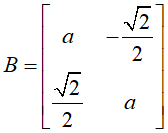1. method: All rotation about origin have matrix form R(Φ), where Φ is rotation angle.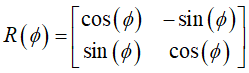Comparing elements of matrices R(Φ) and B yields . 2. method: Rotation is a direct isometry, hence |B|=1, i.e. . 3. method (experimental): Use tool sliderfor unknown parameter a. Define one parameter family of matrices B(a). B={{a,-sqrt(2)/2},{sqrt(2)/2,a}} Draw arbitrary object A (point, segment or picture) and its image A' - GeoGebra command ApplyMatrix(B,A). Observe the effect of changing the slider a and estimate correct value for parameter a. Experimental method is efficient for determination of fixed point and directions. Compare the position of arbitrary movable point A and its image A. Find out the location where points coincide, A = A'. There is the fixed point of transformation. The same method applyed on line f gives you fixed direction. You should find the position where f is parallel with image f'. Fixed points x'=x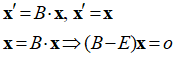where E is identity matrix. First determine substraction BE=B-Identity(2) and than use GeoGebra tool  ReducedRowEchelonForm(BE). This eliminates non diagonal elements by row operations (= Gaussian elimination).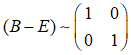Using back-substitution, unknowns x, y can be solved for. Solution x = 0 and y = 0 gives only one fixed point FP = (0,0).

## Fixed directions x'=λx

Eigenvector x of matrix B has invariant direction in transformation defined by matrix B.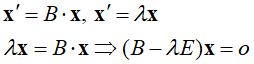Matrix (B-λE) must be singular for non trivial solutions x, but  Det(B-λE)=0  has no real solutin. Rotation has no fixed direction.